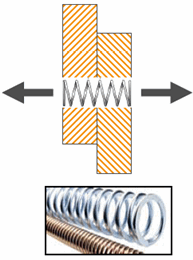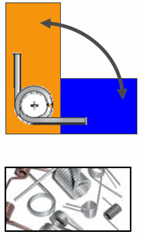Stiffnesses for Pin Connectors

All applicable studies model the spring used for a pin connector as linear elastic. You can estimate the axial and rotational stiffness values based on the actual pin geometry:

 Axial stiffness Rotational stiffnessYou can use the linear stiffness of a rod with constant diameter in tension.K = AE/L A is the cross sectional area of the spring E is the elastic modulus of the spring L is the free length of the spring without any deflection You can use the linear stiffness of rod with constant diameter in torsion.K = JG/L J is the polar moment of inertia = 2I I is the moment of inertia = (Πr4)/4 r is the radius of the coil G is the modulus of rigidity = E/(2(1+ν)) E is the elastic modulus of the spring ν is the Poisson's ratio L is the free length of the spring without any deflection